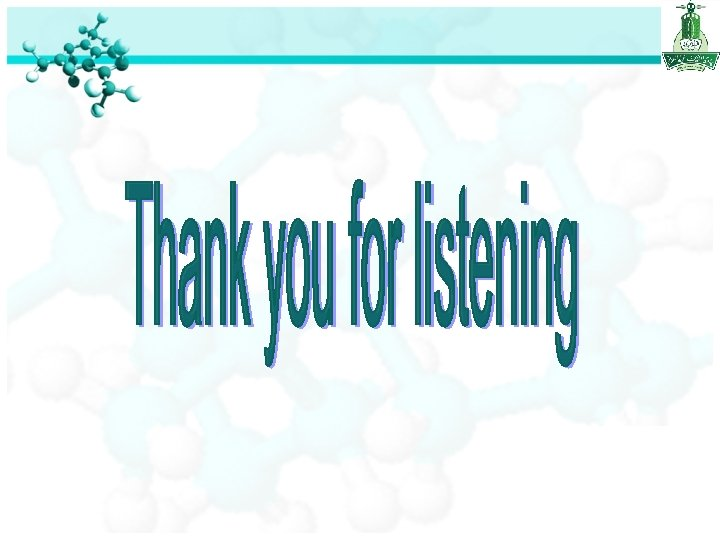Chapter Five Gases Chapter Five Gases Substances That

• Slides: 46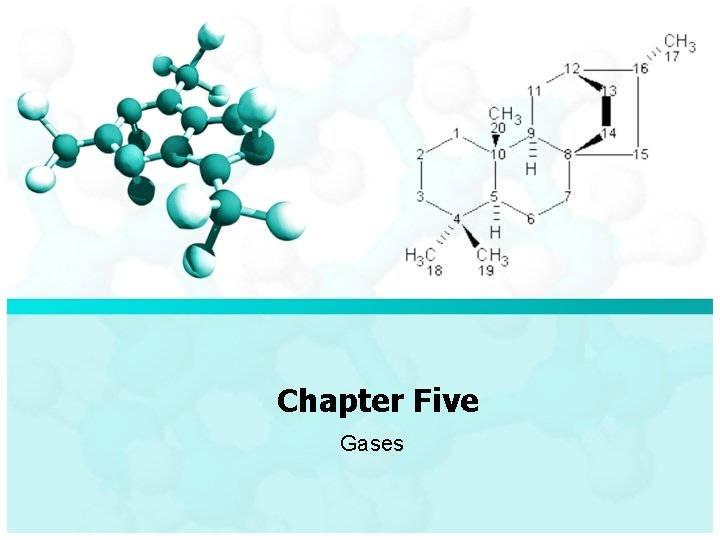Chapter Five Gases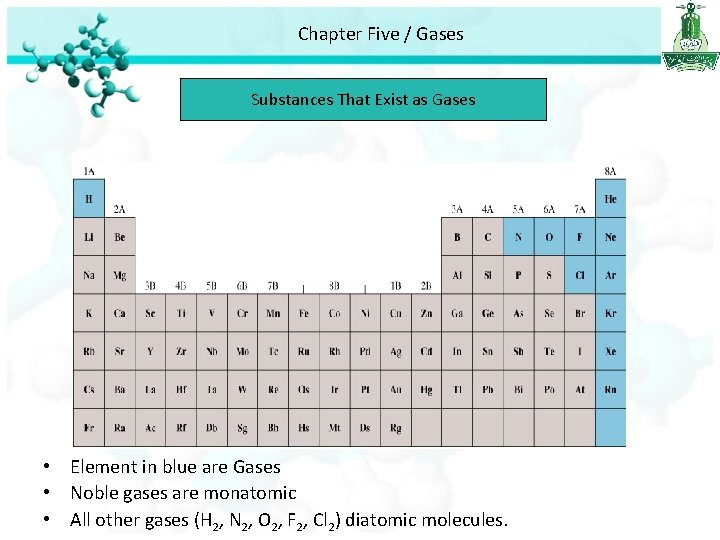Chapter Five / Gases Substances That Exist as Gases • Element in blue are Gases • Noble gases are monatomic • All other gases (H 2, N 2, O 2, F 2, Cl 2) diatomic molecules.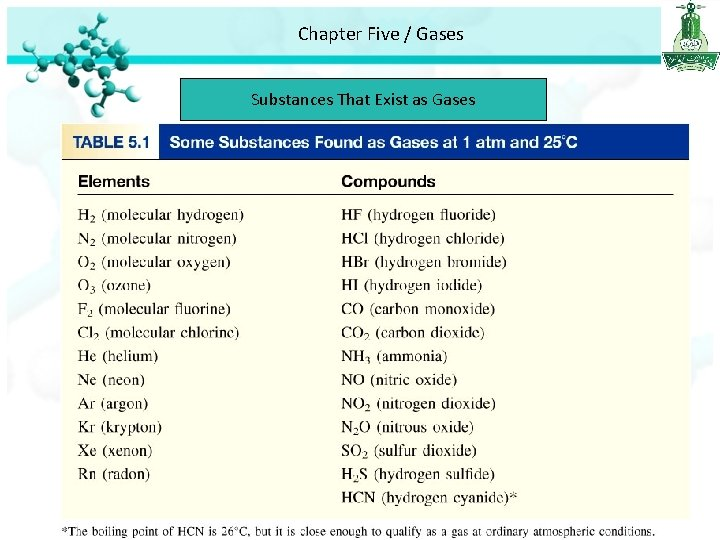Chapter Five / Gases Substances That Exist as GasesChapter Five / Gases Substances That Exist as Gases Physical characteristics of Gases : • Gases assume the volume and shape of their containers. • Gases are the most compressible of the states of matter. • Gases will mix evenly and completely when confined to the same container. • Gases have much lower densities than liquids and solids.Chapter Five / Gases Gas pressure • Gas particles are in constant moving thus they collide with objects in their bath. • The gases push against the walls of their containers with a force. • These collisions produce what we called Gas pressure.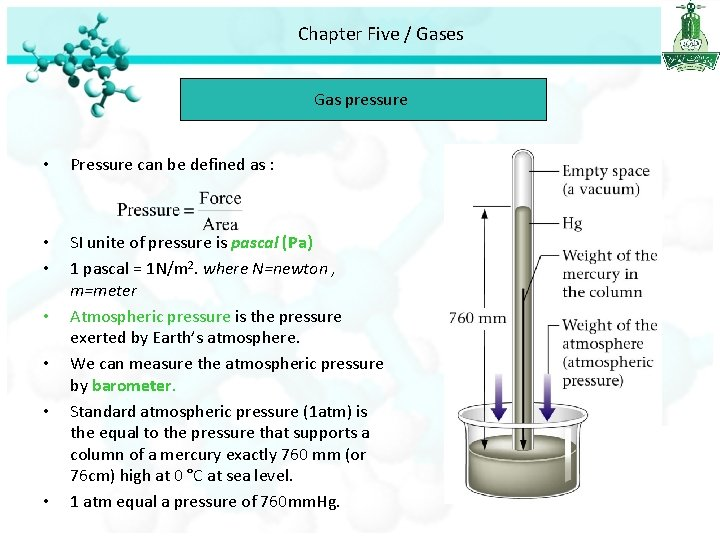Chapter Five / Gases Gas pressure • Pressure can be defined as : • • SI unite of pressure is pascal (Pa) 1 pascal = 1 N/m 2. where N=newton , m=meter Atmospheric pressure is the pressure exerted by Earth’s atmosphere. We can measure the atmospheric pressure by barometer. Standard atmospheric pressure (1 atm) is the equal to the pressure that supports a column of a mercury exactly 760 mm (or 76 cm) high at 0 °C at sea level. 1 atm equal a pressure of 760 mm. Hg. • •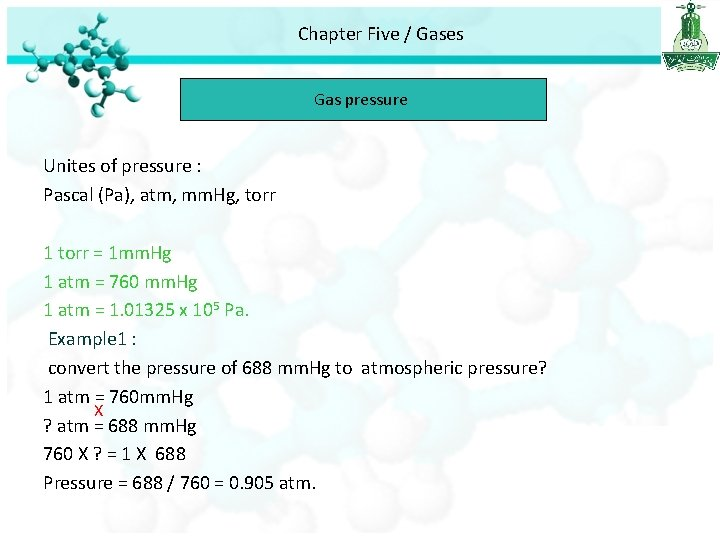Chapter Five / Gases Gas pressure Unites of pressure : Pascal (Pa), atm, mm. Hg, torr 1 torr = 1 mm. Hg 1 atm = 760 mm. Hg 1 atm = 1. 01325 x 105 Pa. Example 1 : convert the pressure of 688 mm. Hg to atmospheric pressure? 1 atm = 760 mm. Hg X ? atm = 688 mm. Hg 760 X ? = 1 X 688 Pressure = 688 / 760 = 0. 905 atm.Chapter Five / Gases Gas pressure Monometer Gas pressure is less than atmospheric pressure Gas pressure is grater than atmospheric pressureChapter Five / Gases Gas Laws • For every gas there are : P (pressure ), T (Temperature) V (volume ), n (mole number). The Pressure – Volume Relationships Boyle’s Law • Boyles’s law study the relationship between the pressure and volume of gas. • Boyel’s law stated that the pressure of a fixed amount of gas at a constant temperature in inversely proportional to the volume of the gas.Chapter Five / Gases Gas Laws- Boyle’s Law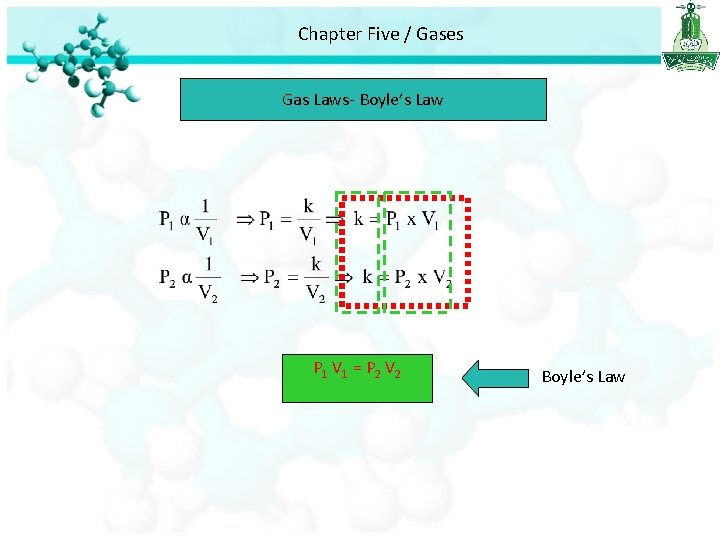Chapter Five / Gases Gas Laws- Boyle’s Law P 1 V 1 = P 2 V 2 Boyle’s LawChapter Five / Gases Gas Laws- Boyle’s Law Example 1 : A sample of chlorine gas occupies a volume of 946 m. L at a pressure of 726 mm. Hg. What is the pressure of the gas (in mm. Hg) if the volume is reduced at constant temperature to 154 m. L? P 1 = 726 mm. Hg, V 1= 946 ml, P 2= ? , V 2= 154 m. L. P 1 V 1 = P 2 V 2 726 x 946 = P 2 x 154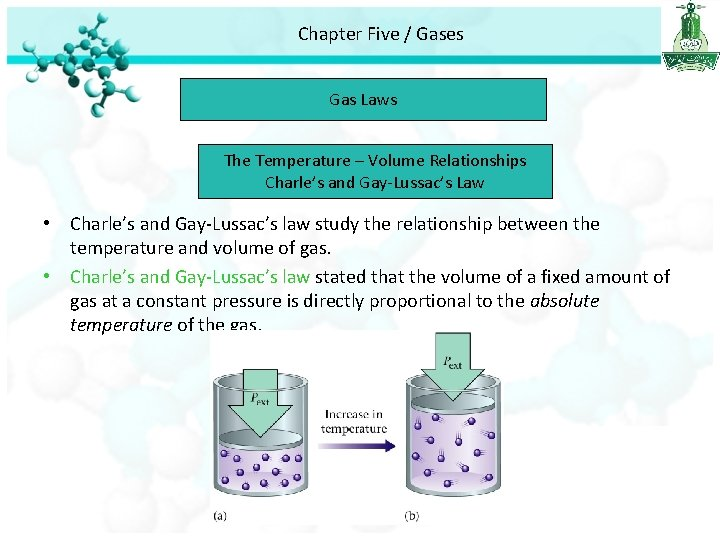Chapter Five / Gases Gas Laws The Temperature – Volume Relationships Charle’s and Gay-Lussac’s Law • Charle’s and Gay-Lussac’s law study the relationship between the temperature and volume of gas. • Charle’s and Gay-Lussac’s law stated that the volume of a fixed amount of gas at a constant pressure is directly proportional to the absolute temperature of the gas.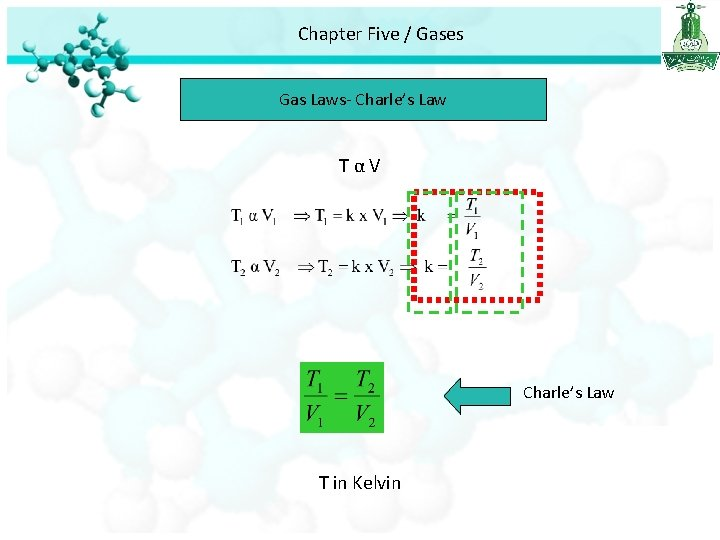Chapter Five / Gases Gas Laws- Charle’s Law TαV Charle’s Law T in KelvinChapter Five / Gases Gas Laws- Charle’s Law Example: A sample of carbon monoxide gas occupies 3. 20 L at 125 °C. At what temperature will the gas occupy a volume of 1. 54 L if the pressure remains constant? T 2 X 3. 2 = 398 X 1. 54 T 2 = 612. 92/3. 2 = 191. 5 K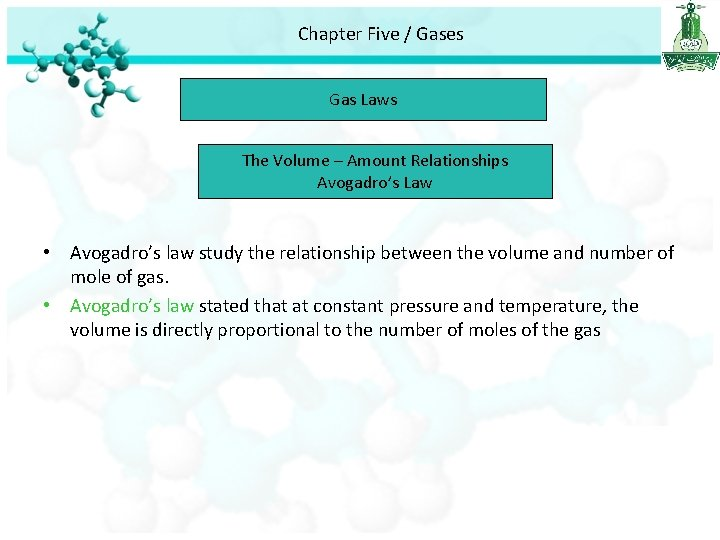Chapter Five / Gases Gas Laws The Volume – Amount Relationships Avogadro’s Law • Avogadro’s law study the relationship between the volume and number of mole of gas. • Avogadro’s law stated that at constant pressure and temperature, the volume is directly proportional to the number of moles of the gas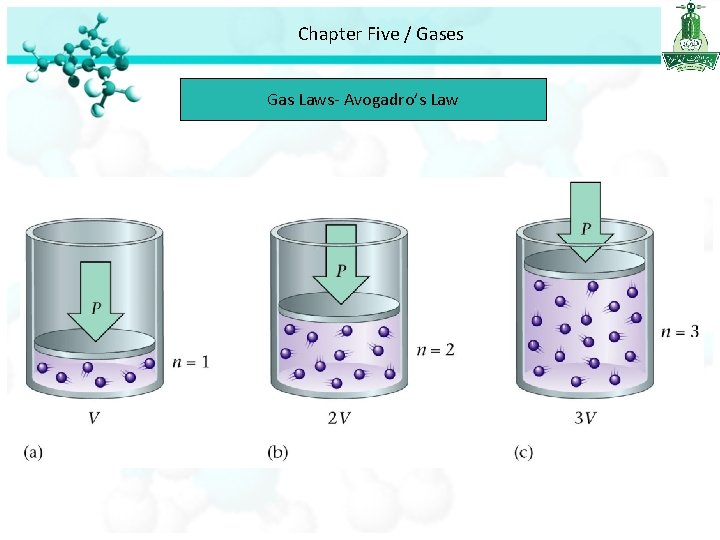Chapter Five / Gases Gas Laws- Avogadro’s LawChapter Five / Gases Summery of Gas Laws P 1 V 1 = P 2 V 2 Boyle’s Law Constant T and n Charle’s Law Constant P and n Avogadro’s Law Constant P and T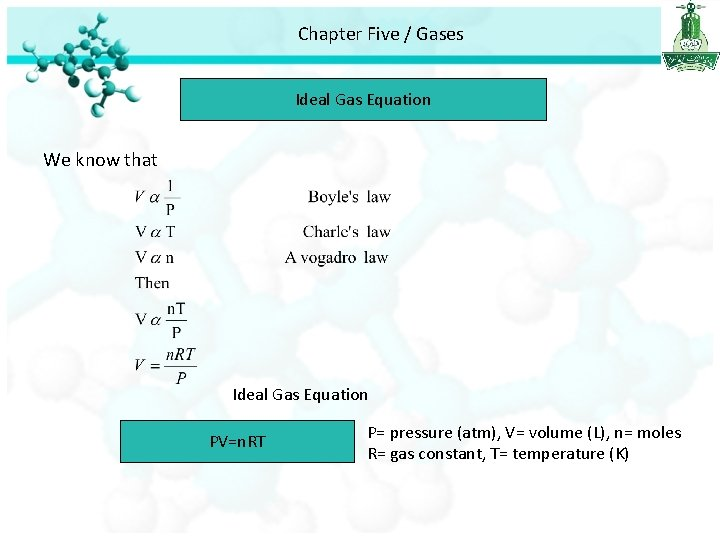Chapter Five / Gases Ideal Gas Equation We know that Ideal Gas Equation PV=n. RT P= pressure (atm), V= volume (L), n= moles R= gas constant, T= temperature (K)Chapter Five / Gases Ideal Gas Equation • Ideal gas is a hypothetical gas whose pressure-volume-temperature behavior can be completely accounted for by the ideal gas equation. • STP : standard Temperature and pressure • Standard Temperature = 0°C = 273. 15 K • Standard Pressure = 1 atm. • At STP 1 mole of an ideal gas occupies 22. 414 L. • R (gas constant ) = 0. 0821 L. atm / K. molChapter Five / Gases Ideal Gas Equation Example 1: Calculate the pressure (in atm) exerted by 1. 82 moles of the sulphur hexaflouride in a steel vessel of volume 5. 43 L at 69. 5 °C. ? PV =n. RT P = n. RT/V = 1. 82 x 0. 0821 x (69. 5 +273)/5. 43 =9. 41 atm.Chapter Five / Gases Ideal Gas Equation Example 2: Calculate the volume (in liters) occupied by 7. 40 g of NH 3 at STP condition. ? PV=n. RT n = mass/molar mass n = 7. 40 / 17 = 0. 435 mol V = n. RT/P V= 0. 435 X 0. 082 X 273 / 1 = 9. 74 L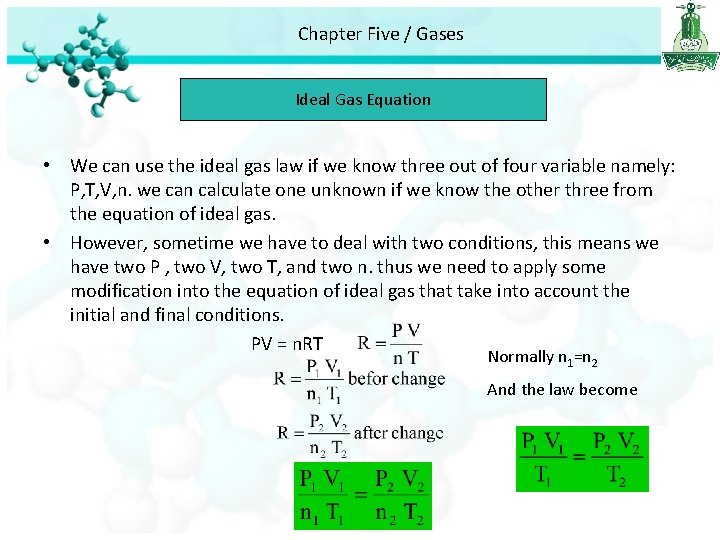Chapter Five / Gases Ideal Gas Equation • We can use the ideal gas law if we know three out of four variable namely: P, T, V, n. we can calculate one unknown if we know the other three from the equation of ideal gas. • However, sometime we have to deal with two conditions, this means we have two P , two V, two T, and two n. thus we need to apply some modification into the equation of ideal gas that take into account the initial and final conditions. PV = n. RT Normally n 1=n 2 And the law become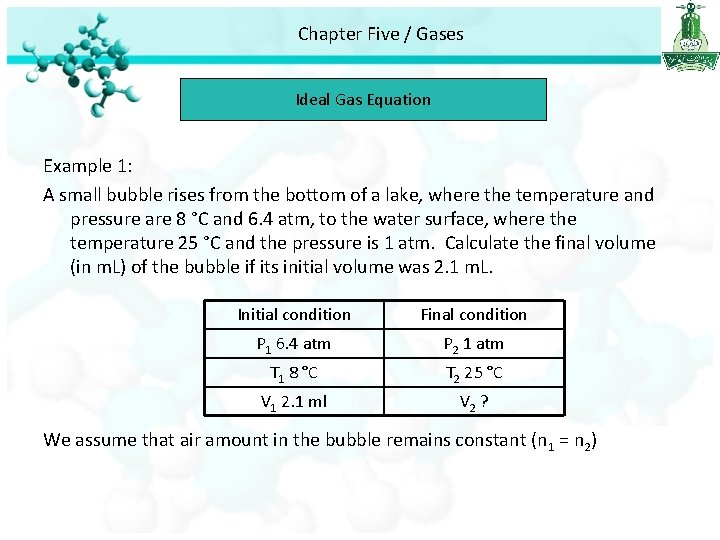Chapter Five / Gases Ideal Gas Equation Example 1: A small bubble rises from the bottom of a lake, where the temperature and pressure are 8 °C and 6. 4 atm, to the water surface, where the temperature 25 °C and the pressure is 1 atm. Calculate the final volume (in m. L) of the bubble if its initial volume was 2. 1 m. L. Initial condition Final condition P 1 6. 4 atm P 2 1 atm T 1 8 °C T 2 25 °C V 1 2. 1 ml V 2 ? We assume that air amount in the bubble remains constant (n 1 = n 2)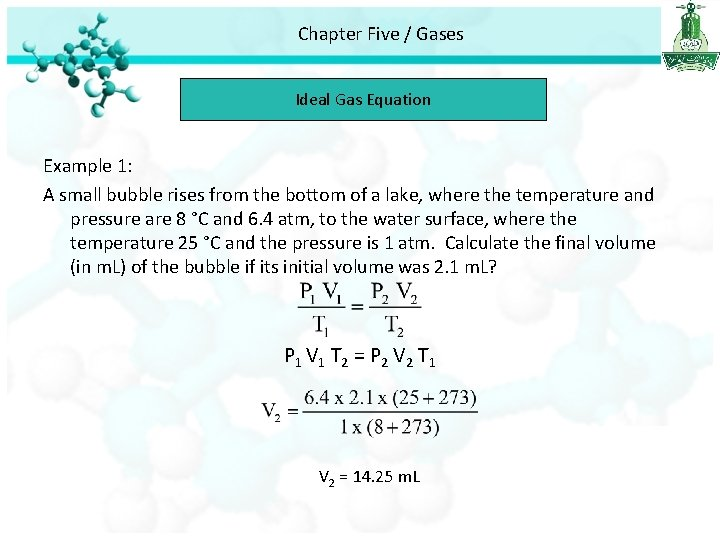Chapter Five / Gases Ideal Gas Equation Example 1: A small bubble rises from the bottom of a lake, where the temperature and pressure are 8 °C and 6. 4 atm, to the water surface, where the temperature 25 °C and the pressure is 1 atm. Calculate the final volume (in m. L) of the bubble if its initial volume was 2. 1 m. L? P 1 V 1 T 2 = P 2 V 2 T 1 V 2 = 14. 25 m. LChapter Five / Gases Ideal Gas Equation Example 2: An inflated helium balloon with a volume of 0. 55 L at sea level (1 atm) is allowed to rise to a high of 6. 5 km. where the pressure is about 0. 40 atm. Assuming that the temperature remains constant. What is the final volume of the balloon? We assume that n 1 = n 2 and T 1 = T 2 P 1 V 1 = P 2 V 2 1 x 0. 55 = 0. 4 x V 2 = 0. 55 / 0. 4 = 1. 4 L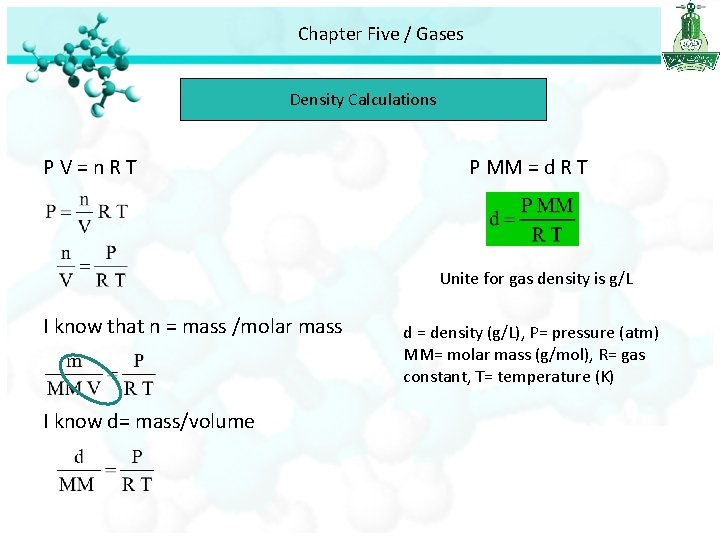Chapter Five / Gases Density Calculations PV=n. RT P MM = d R T Unite for gas density is g/L I know that n = mass /molar mass I know d= mass/volume d = density (g/L), P= pressure (atm) MM= molar mass (g/mol), R= gas constant, T= temperature (K)Chapter Five / Gases Density Calculations Example 1: Calculate the density of CO 2 in g/L at 0. 990 atm and 55 °C? MM(CO 2)= 40 g/mol d= 1. 47 g/L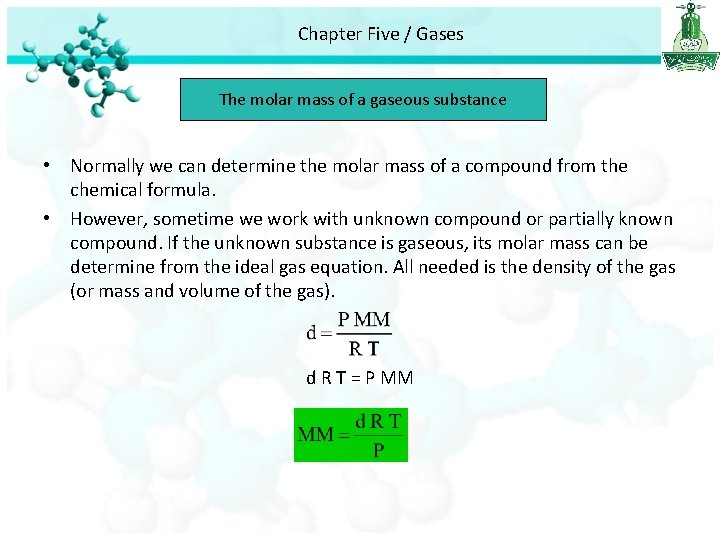Chapter Five / Gases The molar mass of a gaseous substance • Normally we can determine the molar mass of a compound from the chemical formula. • However, sometime we work with unknown compound or partially known compound. If the unknown substance is gaseous, its molar mass can be determine from the ideal gas equation. All needed is the density of the gas (or mass and volume of the gas). d R T = P MM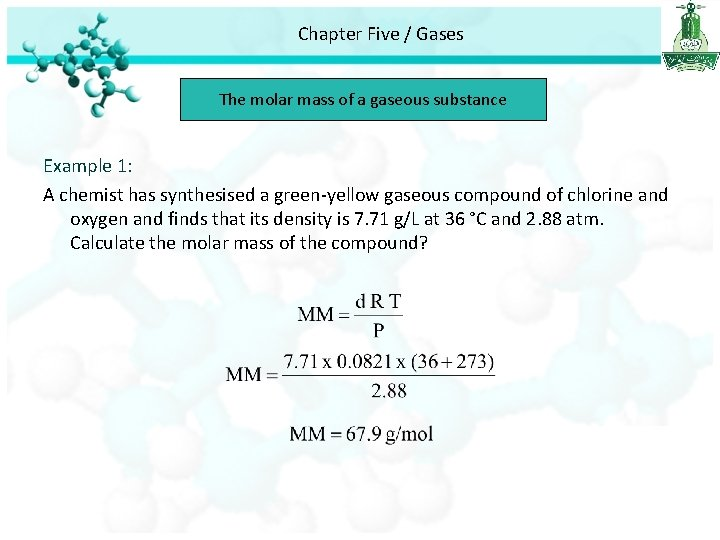Chapter Five / Gases The molar mass of a gaseous substance Example 1: A chemist has synthesised a green-yellow gaseous compound of chlorine and oxygen and finds that its density is 7. 71 g/L at 36 °C and 2. 88 atm. Calculate the molar mass of the compound?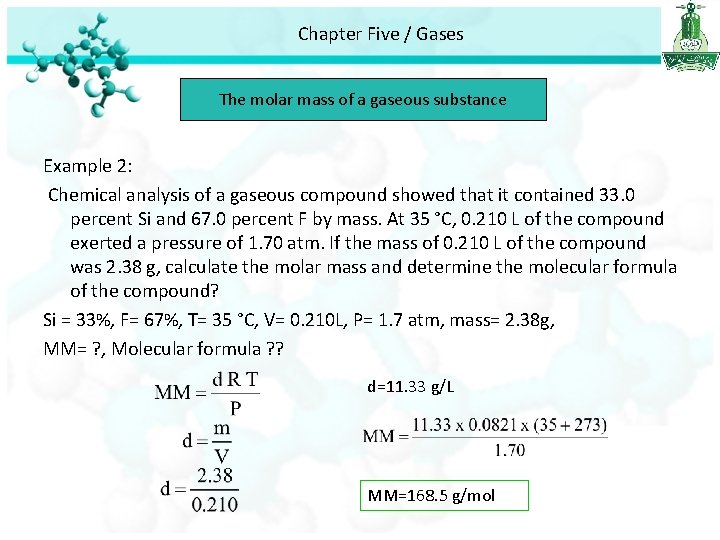Chapter Five / Gases The molar mass of a gaseous substance Example 2: Chemical analysis of a gaseous compound showed that it contained 33. 0 percent Si and 67. 0 percent F by mass. At 35 °C, 0. 210 L of the compound exerted a pressure of 1. 70 atm. If the mass of 0. 210 L of the compound was 2. 38 g, calculate the molar mass and determine the molecular formula of the compound? Si = 33%, F= 67%, T= 35 °C, V= 0. 210 L, P= 1. 7 atm, mass= 2. 38 g, MM= ? , Molecular formula ? ? d=11. 33 g/L MM=168. 5 g/mol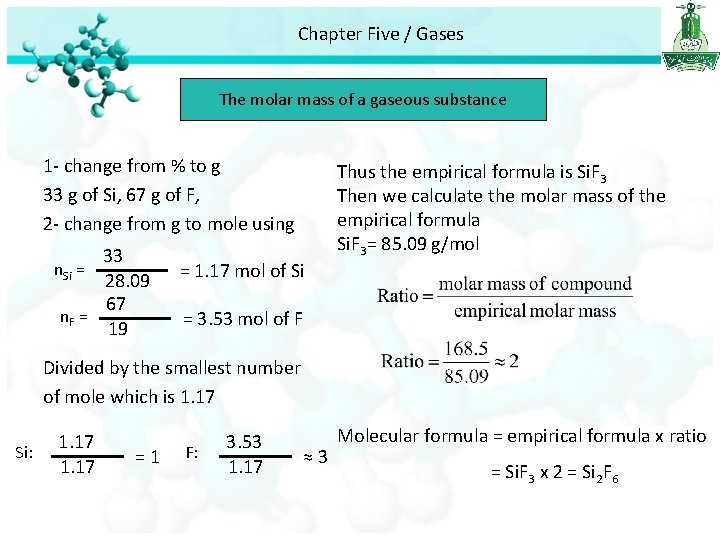Chapter Five / Gases The molar mass of a gaseous substance 1 - change from % to g 33 g of Si, 67 g of F, 2 - change from g to mole using 33 28. 09 67 n. F = 19 n. Si = = 1. 17 mol of Si Thus the empirical formula is Si. F 3 Then we calculate the molar mass of the empirical formula Si. F 3= 85. 09 g/mol = 3. 53 mol of F Divided by the smallest number of mole which is 1. 17 Si: 1. 17 =1 F: 3. 53 1. 17 ≈3 Molecular formula = empirical formula x ratio = Si. F 3 x 2 = Si 2 F 6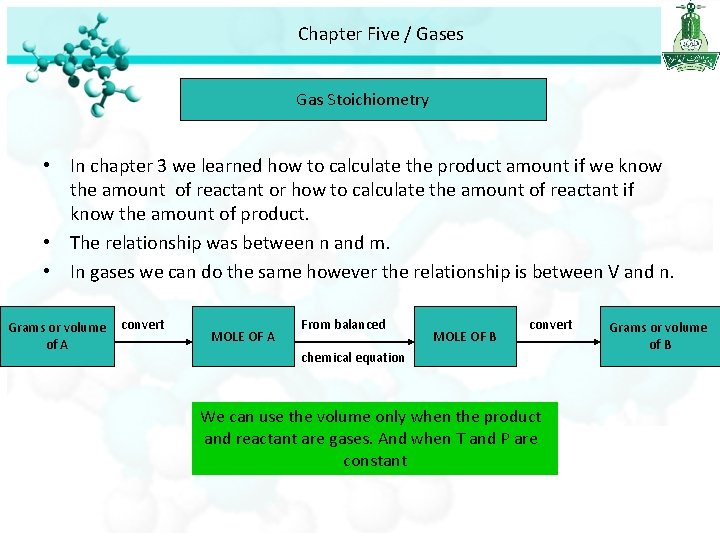Chapter Five / Gases Gas Stoichiometry • In chapter 3 we learned how to calculate the product amount if we know the amount of reactant or how to calculate the amount of reactant if know the amount of product. • The relationship was between n and m. • In gases we can do the same however the relationship is between V and n. Grams or volume of A convert MOLE OF A From balanced MOLE OF B convert chemical equation We can use the volume only when the product and reactant are gases. And when T and P are constant Grams or volume of BChapter Five / Gases Gas Stoichiometry Example 1: Calculate the volume of O 2 (in L) required for the complete combustion of 7. 64 L of C 2 H 2 measured at the same temperature and pressure. ? From equation 2 mol C 2 H 2 5 mol O 2 2 L C 2 H 2 5 L O 2 7. 64 L C 2 H 2 X ? L O 2 5 x 7. 64 = 2 x ? Volume of O 2 = 5 X 7. 64 / 2 = 19. 1 LChapter Five / Gases Gas Stoichiometry Example 2: Sodium azide (Na. N 3) is used in some automobile air bags. The impact of a collision triggers the decomposition of Na. N 3 as follows: The nitrogen gas produced quickly inflates the bag between the driver and the windshield and dashboard. Calculate the volume of N 2 generated at 80 °C and 823 mm. Hg by the decomposition of 60 g Na. N 3? T = 80 °C = 80 +273 = 353 K P= 823 mm. Hg 1 atm =760 mm. Hg ? atm = 823 mm. Hg From equation 823 x 1 = 760 x ? = 823/760 =1. 083 atm 2 mol Na. N 3 3 mol N 2 m= 60 g. X 0. 923 mol Na. N 3 ? mol N 2 First convert g to mole 3 x 0. 923 = 2 x ? n=m/MM Mole of N 2= 3 x 0. 923 / 2 = 60 / 65. 02 =0. 923 mol Mole of N 2 = 1. 38 molChapter Five / Gases Gas Stoichiometry PV = n. RT V = 36. 9 L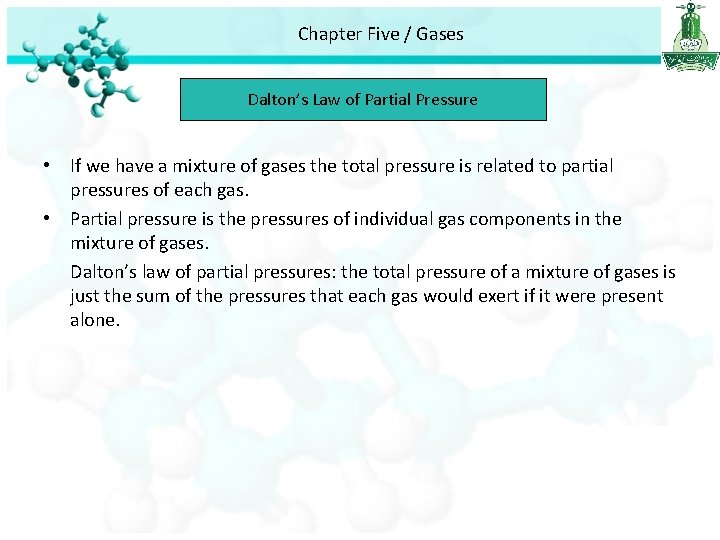Chapter Five / Gases Dalton’s Law of Partial Pressure • If we have a mixture of gases the total pressure is related to partial pressures of each gas. • Partial pressure is the pressures of individual gas components in the mixture of gases. Dalton’s law of partial pressures: the total pressure of a mixture of gases is just the sum of the pressures that each gas would exert if it were present alone.Chapter Five / Gases Dalton’s Law of Partial Pressure V and T are constant P 1 P 2 Ptotal = P 1 + P 2Chapter Five / Gases Dalton’s Law of Partial Pressure PT = P A + PB Where n = n. A + n. B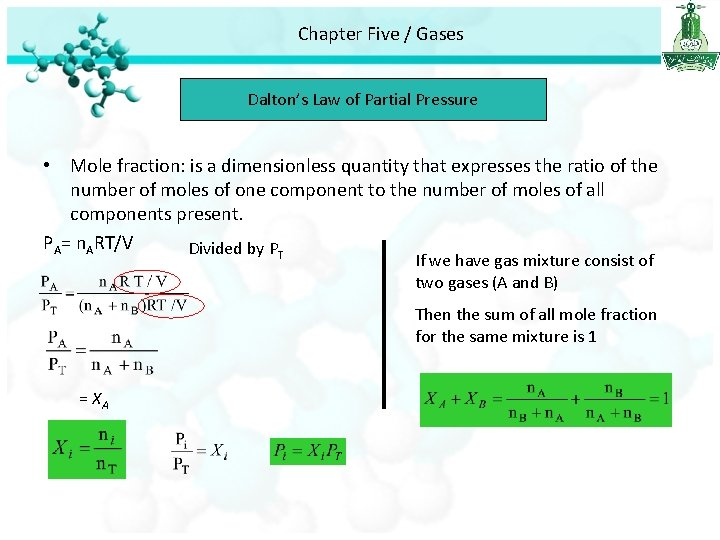Chapter Five / Gases Dalton’s Law of Partial Pressure • Mole fraction: is a dimensionless quantity that expresses the ratio of the number of moles of one component to the number of moles of all components present. PA= n. ART/V Divided by PT If we have gas mixture consist of two gases (A and B) Then the sum of all mole fraction for the same mixture is 1 = XA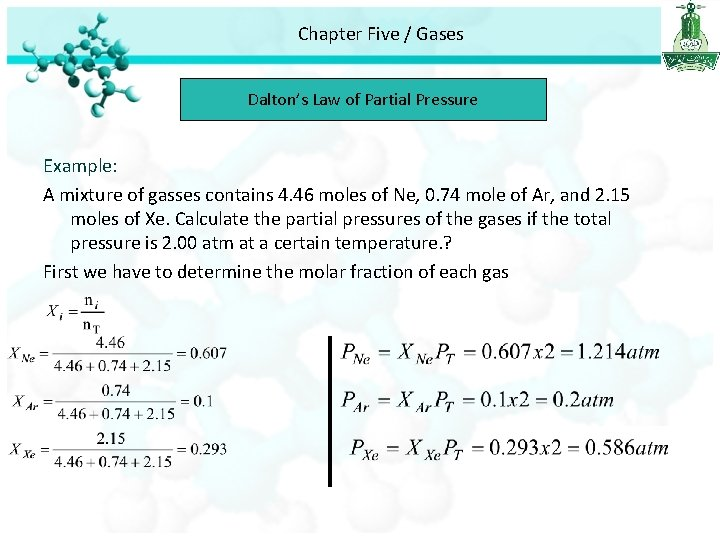Chapter Five / Gases Dalton’s Law of Partial Pressure Example: A mixture of gasses contains 4. 46 moles of Ne, 0. 74 mole of Ar, and 2. 15 moles of Xe. Calculate the partial pressures of the gases if the total pressure is 2. 00 atm at a certain temperature. ? First we have to determine the molar fraction of each gas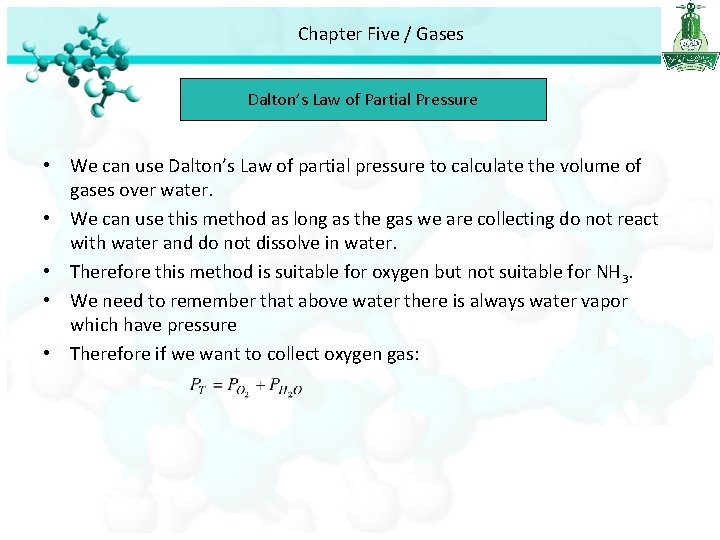Chapter Five / Gases Dalton’s Law of Partial Pressure • We can use Dalton’s Law of partial pressure to calculate the volume of gases over water. • We can use this method as long as the gas we are collecting do not react with water and do not dissolve in water. • Therefore this method is suitable for oxygen but not suitable for NH 3. • We need to remember that above water there is always water vapor which have pressure • Therefore if we want to collect oxygen gas:Chapter Five / Gases Dalton’s Law of Partial Pressure Example: Oxygen gas generated by the decomposition of potassium chlorate is collected. The volume of oxygen collected at 24 °C and atmospheric pressure of 762 mm. Hg is 128 ml. Calculate the mass (in g) of oxygen gas obtained. The pressure of the water vapour at 24 °C is 22. 4 mm. Hg. ? T=24 °C = 24 + 273 = 297 K, VO 2 =128 ml = 128/1000=0. 128 L , m = ? ? PT = 762 mm. Hg, 1 atm = 760 mm. Hg ? atm = 762 mm. Hg 762 x 1= ? x 760 762 /760 =1. 002 atm PH 20=22. 4 mm. Hg, 1 atm = 760 mm. Hg ? atm = 22. 4 mm. Hg 22. 4 x 1= ? x 760 22. 4 /760 =0. 029 atm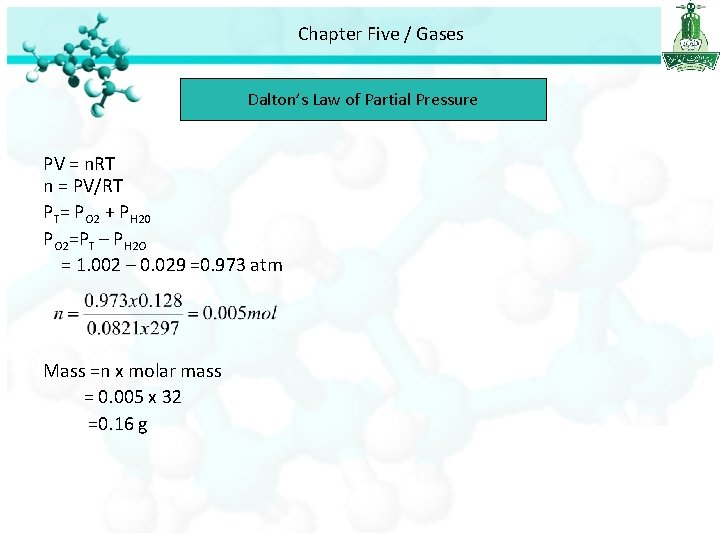Chapter Five / Gases Dalton’s Law of Partial Pressure PV = n. RT n = PV/RT PT= PO 2 + PH 20 PO 2=PT – PH 2 O = 1. 002 – 0. 029 =0. 973 atm Mass =n x molar mass = 0. 005 x 32 =0. 16 g